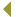THE UNIVERSITY OF GEORGIA RESEARCH MAGAZINE : www.researchmagazine.uga.eduBACK TO WEB VERSION FALL 2005 Knotty Problem Solved by Philip Lee Williams and Carole VanSickle It’s not as famous as Fermat’s Last Theorem, which took 350 years to solve. It doesn’t even have a name. But Dino Lorenzini, a mathematics professor at the University of Georgia, and two French colleagues, Michel Raynaud and Qing Liu, recently devised an elegant solution to a 40-year-old problem about understanding arithmetically interesting points on a curve. The solution is published in a two-part paper in the September 2004 and March 2005 issues of Inventiones Mathematicae, one of the world’s top math journals. Such intractable challenges in mathematics have long held allure. That it took more than three centuries for mathematicians to solve Fermat’s Last Theorem is a testament to the difficulty of solving a problem involving curves and integer solutions. To study this more recent one, mathematicians associated to the curve two special groups: group A (the Shafarevich-Tate group) and group B (the Brauer group). Mathematical groups come in all sorts of shapes and sizes. They lie at the heart of attempts to classify what is going on in the world of numbers. They also may be applied to chemistry, physics and just about any other subject that uses math. About 10 years after the knotty, unnamed problem was determined, mathematicians decided that the number of elements in group A could only be a perfect square (such as 1, 4, 9, 16…), while the number of elements in group B wasn’t always a square. These views held for 30 years until Lorenzini’s team proved them wrong. The trio of mathematicians found a precise formula relating the sizes of groups A and B, and used it to show that the size of group B is also always a square, but also demonstrating the number of elements in group A — the total number in the group — isn’t always a square. “What makes the story interesting is the twist,” said Lorenzini. “Seldom are two major assumptions corrected after only 30 years. But the real issue is that the groups A and B are so important that any advance in the area is of interest.” For more information, e-mail Dino Lorenzini at lorenzini@math.uga.edu. THE UNIVERSITY OF GEORGIA RESEARCH MAGAZINE : www.researchmagazine.uga.edu